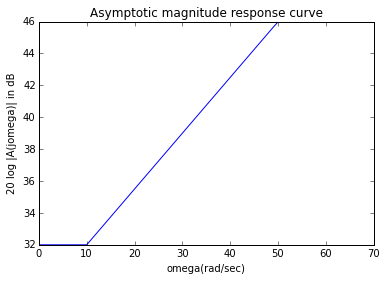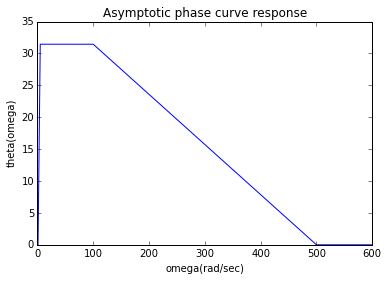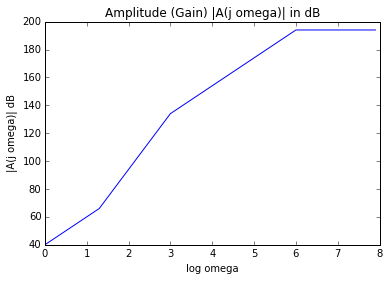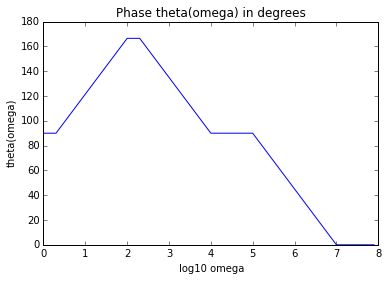# Chapter 10 : Frequency response of amplifiers¶

## Example 10.1: Page No 450¶

In :
from __future__ import division
from scipy import log10
from numpy import nditer
import numpy as np
% matplotlib inline
from matplotlib.pyplot import plot, title, xlabel, ylabel, show

#Asymptotic magnitude and phase response curves
w=range(0,70)
# Asymptotic magnitude response curve
a=[]
b=[]
c=[]
for i in range(0,len(w)):
a.append(32)
if w[i]<10:
b.append(0)
c.append(0)
elif w[i]<50:
b.append(14*(w[i]-10)/40)
c.append(0)
else:
b.append(20*log10(w[i]/10))
c.append(-20*log10(w[i]/50))

A=[]
for x,y,z in nditer([a,b,c]):
A.append(x+y+z)
#A=a+b+c#

plot(w,A)
title("Asymptotic magnitude response curve")
ylabel("20 log |A(jomega)| in dB")
show()
# Asymptotic phase response curve
w=range(0,600)
theta1=[]
theta2=[]
for i in range(0,len(w)):
if w[i]<1:
theta1.append(0)
theta2.append(0)
elif w[i]<5:
theta1.append(31.45*(w[i]-1)/4)
theta2.append(0)
elif w[i]<100:
theta1.append(45*log10(w[i]/10))
theta2.append(-45*log10(w[i]/50))
elif w[i]<500:
theta1.append(90)
theta2.append(-58.55-31.45*(w[i]-100)/400)
else:
theta1.append(90)
theta2.append(-90)

theta=[]
for x,y in nditer([theta1,theta2]):
theta.append(x+y)

plot(w,theta)#
title("Asymptotic phase curve response")
ylabel("theta(omega)")
show()## Example 10.2: Page No 453¶

In :
#Bode's plots
from numpy import arange,nditer
%matplotlib inline
from matplotlib.pyplot import plot, title, show, xlabel, ylabel

w=arange(0,8,0.1)
# Asymptotic magnitude response curve
a=[]
b=[]
c=[]
d=[]
e=[]
for i in range(0,len(w)):
a.append(40)
if w[i]<1.3:
b.append(20*w[i])
c.append(0)
d.append(0)
e.append(0)
elif w[i]<3:
b.append(20*w[i])
c.append(20*(w[i]-1.3))
d.append(0)
e.append(0)
elif w[i]<6:
b.append(20*w[i])
c.append(20*(w[i]-1.3))
d.append(-20*(w[i]-3))
e.append(0)
else:
b.append(20*w[i])
c.append(20*(w[i]-1.3))
d.append(-20*(w[i]-3))
e.append(-20*(w[i]-6))

A=[]

for m,n,o,p,q in nditer([a, b, c, d, e]):
A.append(m+n+o+p+q)

plot(w,A)
title("Amplitude (Gain) |A(j omega)| in dB")
xlabel("log omega")
ylabel("|A(j omega)| dB")
show()
# Asymptotic phase response curve

thetab=[]
thetac=[]
thetae=[]
for i in range(0,len(w)):
thetab.append(90)
if w[i]<0.3:
thetac.append(0)
thetae.append(0)
elif w[i]<2:
thetac.append(45*(w[i]-0.3))
thetae.append(0)
elif w[i]<2.3:
thetac.append(45*(w[i]-0.3))
thetae.append(0)
elif w[i]<4:
thetac.append(90)
thetae.append(0)
elif w[i]<5:
thetac.append(90)
thetae.append(0)
elif w[i]<7:
thetac.append(90)
thetae.append(-45*(w[i]-5))
else:
thetac.append(90)
thetae.append(-90)
theta =[]
for m,n,o,p in nditer([thetab, thetac, thetad, thetae]):
theta.append(m+n+o+p)

plot(w,theta)#
title("Phase theta(omega) in degrees")
xlabel("log10 omega")
ylabel("theta(omega)")
show()## Example 10.3: Page No 461¶

In :
from math import sqrt, pi
#CS, Zero frequency

gm=1e-3# # in mho
fL=10# # in hertz
# From Fig. 10.10
RS=6e3# # in ohms
I=RS/(1+RS*gm)# # Impedance seen by CS in ohms
print "CS = %0.2f µF "%CS
print "Here at f = 0 Hz, CS has infinite reactance."
print "Therefore, zero frequency fzero = 0 Hz here, i.e. the voltage transfer function is zero at DC."

CS = 18.57 µF
Here at f = 0 Hz, CS has infinite reactance.
Therefore, zero frequency fzero = 0 Hz here, i.e. the voltage transfer function is zero at DC.


## Example 10.4: Page No 475¶

In :
from math import sqrt,pi
#fT, fb

b_o=160#
f=50# # in Mega-hertz
b_jw=8#
fb=wb/(2*pi)# # in Mega-hertz
fT=fb*b_o# # in Mega-hertz
print "fT = %0.2f MHz "%fT
print "fb = %0.2f MHz "%fb

fT = 400.50 MHz
fb = 2.50 MHz


## Example 10.5: Page No 477¶

In :
from math import sqrt,pi
#Cπ

IC=1e-3# # in amperes
b_o=120#
b_jw=10#
f=25e6# # in hertz
VT=25e-3# # Voltage equivalent to temperatue at room temperature in volts
wT=wb*b_o# # in hertz
gm=IC/VT# # in mho
print "Cπ = %0.2f pF "%C_pi

Cπ = 24.38 pF


## Example 10.7: Page No 490¶

In :
from math import sqrt,pi
#               (a) Midband gain, Upper half-power frequency
#               (b) Zi

ICQ=1e-3# # in amperes
RS=300# # in ohms
RC=1.2e3# # in ohms
bta=125#
fT=300e6# # in hertz
VT=25e-3# # Voltage equivalent to temperatue at room temperature in volts

print "Part (a)"
gm=ICQ/VT# # in mho
r_pi=bta/gm# # in ohms
# To find C_pi
AVo=-bta*RC/(RS+r_pi)# # Midband gain
print "Midband gain = %0.2f "%AVo
R_pi0=RS*r_pi/(RS+r_pi)#
a1=R_pi0*C_pi+(R_pi0+RC*(1+gm*R_pi0))*C_mu# # in seconds
a2=R_pi0*RC*C_pi*C_mu# # in seconds
print "p2/p1 = %0.2f "%(p2/p1)
print "Since p2/p1 >> 8, therefore dominant-pole approximation holds good."
print "Upper half-power frequency = %0.2f M rad/sec "%wH

print "Part (b)"
Zi=r_pi/(1+1J*wH*1e6*CM*r_pi)# # in ohms
print "Zi ={0:.2f}+{1:.2f}j".format(Zi.real,Zi.imag),"Ω "

Part (a)
Midband gain = -43.80
p2/p1 = 49.49
Since p2/p1 >> 8, therefore dominant-pole approximation holds good.
Upper half-power frequency = 77.05 M rad/sec
Part (b)
Zi =26.14+-284.59j Ω


## Example 10.12: Page No 530¶

In :
from math import sqrt,pi
#                (a) Approximate value of fH
#                (b) Approximate location of the closest non-dominant pole

RS=600# # in ohms
RC1=1.5e3# # in ohms
RC2=600# # in ohms
r_pi1=1.2e3# # in ohms
gm1=0.1# # in mho
r_pi2=2.4e3# # in ohms
gm2=0.05# # in mho
def parallel(a,b):
c=a*b/(a+b)
return c

print "Part (a)"
R11_0=parallel(RS,r_pi1)# # in ohms
R33_0=parallel(RC1,r_pi2)# # in ohms
R22_0=R11_0*(1+gm1*R33_0)+R33_0# # in ohms
R44_0=R33_0*(1+gm2*RC2)+RC2# # in ohms
a1=R11_0*C1+R22_0*C2+R33_0*C3+R44_0*C4# # in seconds
fH=1/(2*pi*a1)# # in hertz
fH=fH*1e-6# # in Mega-hertz
print "fH = %0.2f MHz "%fH

print "Part (b)"
R33_1=R33_0# # in ohms
R44_1=R44_0# # in ohms
# From Fig. 10.61(a)
R22_1=R33_0# # in ohms
# From Fig. 10.61(b)
R44_3=RC2# # in ohms
# From Fig. 10.61(c)
R33_2=parallel(parallel(r_pi2,RC2),parallel(1/gm1,R11_0))#
R44_2=R33_2*(1+gm2*RC2)+RC2# # in ohms
a2=R11_0*C1*R22_1*C2+R11_0*C1*R33_1*C3+R11_0*C1*R44_1*C4+R22_0*C2*R33_2*C3+R22_0*C2*R44_2*C4+R33_0*C3*R44_3*C4# # in seconds
p2=a1/a2#
f2=p2/(2*pi)# # in hertz
f2=f2*1e-6# # in Mega-hertz
print "Approximate location of the closest non-dominant pole = %0.2f MHz "%f2

Part (a)
fH = 2.59 MHz
Part (b)
Approximate location of the closest non-dominant pole = 28.67 MHz


## Example 10.13: Page No 537¶

In :
from math import sqrt,pi
#                (a) fH for cascode amplifier
#                (b) fH for common -emitter stage

RC1=1.5e3# # in ohms
RC2=RC1#
RS=300# # in ohms
r_pi=2e3# # in ohms
gm=0.05# # in mho
bta=100#

print "Part (a)"
R_pi1=RS*r_pi/(RS+r_pi)# # in ohma
Ri2=r_pi/(1+bta)# # in ohms
RL1=RC1*Ri2/(RC1+Ri2)# # in ohms
a11=R_pi1*C_pi+(R_pi1*(1+gm*RL1)+RL1)*C_mu# # in seconds
a12=C_pi/gm+C_mu*RC2# # in seconds
a1=a11+a12# # in seconds
fH=1/(2*pi*a1)# # in hertz
fH=fH*1e-6# # in Mega-hertz
print "fH for cascode amplifier = %0.2f MHz "%fH

print "Part (b)"
a1=R_pi1*C_pi+(R_pi1*(1+gm*RC1)+RC1)*C_mu# # in seconds
fH=1/(2*pi*a1)# # in hertz
fH=fH*1e-6# # in Mega-hertz
print "fH for common-emitter stage = %0.2f MHz "%fH

Part (a)
fH for cascode amplifier = 24.51 MHz
Part (b)
fH for common-emitter stage = 10.11 MHz


## Example 10.15: Page No 548¶

In :
from math import sqrt,pi
##                (a) CB and CL
#                (b) Zero introduced by CE

RE=1.5e3# # in ohms
Rs=600# # in ohms
bta=100#
r_pi=1e3# # in ohms
fL=50# # in hertz

print "Part (a)"
fLB=fL/2# # in hertz
fLE=fLB# # in hertz
def parallel(a,b):
c=a*b/(a+b)
return c
print "CB = %0.2f µF "%CB
print "CE = %0.2f µF "%CE

print "Part (b)"
fE=1e6/(2*pi*RE*CE)# # in hertz
print  "fE = %0.2f Hz "%fE

Part (a)
CB = 3.98 µF
CE = 406.11 µF
Part (b)
fE = 0.26 Hz


## Example 10.16: Page No 549¶

In :
from math import sqrt,pi
#AVo, fH

RC=1.5e3# # in ohms
Rs=0.6e3# # in ohms
# From Fig. 10.69
r_pi=1e3# # in ohms
gm=0.1# # in mho
bta=r_pi*gm#
AVo=-bta*RC/(Rs+r_pi)#
R_pi=Rs*r_pi/(Rs+r_pi)# # in ohms
R_mu=R_pi+(1+gm*R_pi)*RC# # in ohms
a1=R_pi*C_pi+R_mu*C_mu# # in seconds
a2=R_pi*C_pi*R_mu*C_mu# # in seconds
p2_pi=a1**2/a2# # p2/p1
print "Since p2/pi >> 8, therefore dominant-pole approximation holds good."
fH=1/(2*pi*a1)# # in hertz
fH=fH*1e-6# # in Mega-hertz
print "AVo = %0.2f "%AVo
print "fH = %0.2f MHz "%fH

Since p2/pi >> 8, therefore dominant-pole approximation holds good.
AVo = -93.75
fH = 4.38 MHz


## Example 10.17: Page No 550¶

In :
from math import sqrt,pi
#(b) a1, a2

RS=0.3e3# # in ohms
r_pi=2e3# # in ohms
RC=0.6# # in ohms
gm=0.1e-3# # in mho
R_pi=RS*r_pi/(RS+r_pi)# # in ohms
a1=C_pi*R_pi+C_mu*(R_pi+RC+gm*R_pi*RC)# # in seconds
a1=a1*1e9# # in nano-seconds
a2=C_pi*R_pi*C_mu*RC# # in seconds square
print "a1 = %0.2f ns "%a1
print "a2 = %0.2e sec square "%a2

a1 = 5.22 ns
a2 = 1.53e-21 sec square


## Example 10.18: Page No 552¶

In :
from math import sqrt,pi
#Upper 3 dB frequency

r_pi1=1.4e3# # in ohms
r_pi2=2.8e3# # in ohms
gm1=0.15# # in mho
gm2=0.05# # in mho
bta1=gm1*r_pi1#
bta2=gm2*r_pi2#
# From Fig. 10.71
RS=600# # in ohms
RC1=1.5e3# # in ohms
RL2=600# # in ohms
# From ac model in Fig. 10.72
R_pi1=RS*r_pi1/(RS+r_pi1)# # in ohms
RL1=RC1*r_pi2/(RC1+r_pi2)# # in ohms
R_mu1=R_pi1+RL1+gm1*RL1*R_pi1# # in ohms
R_pi2=RL1# # in ohms
R_mu2=R_pi2+RL2+gm2*RL2*R_pi2# # in ohms
a11=C_pi1*R_pi1+C_mu1*R_mu1# # in seconds
a12=C_pi2*R_pi2+C_mu2*R_mu2# # in seconds
a1=a11+a12# # in seconds
fH1=1/(2*pi*a11)# # in hertz
fH2=1/(2*pi*a12)# # in hertz
fH=1/(2*pi*a1)# # in hertz
fH1=fH1*1e-6# # in Mega-hertz
fH2=fH2*1e-6# # in Mega-hertz
fH=fH*1e-6# # in Mega-hertz
AV1=-bta1*RC1/(RS+r_pi1)# # Gain of first stage
AV2=-bta2*RL2/(RC1+r_pi2)# # Gain of second stage
print "Upper 3 dB frequency = %0.2f MHz "%fH
print "Bandwidth:"
print "Stage 1 only = %0.2f MHz "%fH1
print "Stage 2 only = %0.2f MHz "%fH2
print "Cascade = %0.2f MHz "%fH
print "Gain:"
print "Stage 1 only = %0.2f "%abs(AV1)
print "Stage 2 only = %0.2f "%abs(AV2)
print "Gain-bandwidth product:"
print "Stage 1 only = %0.2f MHz "%(fH1*abs(AV1)*1e6)
print "Stage 2 only = %0.2f MHz "%(fH2*abs(AV2)*1e6)
print "Cascade = %0.2f MHz "%(fH*AV*1e6)

Upper 3 dB frequency = 2.00 MHz
Bandwidth:
Stage 1 only = 3.99 MHz
Stage 2 only = 3.99 MHz
Gain:
Stage 1 only = 157.50
Stage 2 only = 19.53
Gain-bandwidth product:
Stage 1 only = 628781932.47 MHz
Stage 2 only = 78003472.90 MHz


## Example 10.19: Page No 554¶

In :
from math import sqrt,pi
#Approximate value of fH

btaf=150#
VA=120# # in volts
fT=400e6# # in hertz
ICQ=100e-6# # in amperes
RS=50e3# # in ohms
RC=250e3# # in ohms
VT=25e-3# # Voltage equivalent to temperatue at room temperature in volts
gm=ICQ/VT# # in mho
r_pi=btaf/gm#  # in ohms
ro=VA/ICQ# # in ohms
def parallel(a,b):
c=a*b/(a+b)#
return c
# From AC model in Fig. 10.73
Ri=r_pi+(1+btaf)*parallel(ro,r_pi)# # in ohms
R_mu1=parallel(RS,Ri)# # in ohms
# From Fig. 10.75(b)
R=(50+36.36)/(1+145)# # in ohms
R_pi1=parallel(r_pi,R)# # in ohms
R_pi2=parallel(r_pi,parallel((RS+r_pi)/(1+btaf),ro))# # in ohms
RL=parallel(ro,RC)# # in ohms
R_mu2=R_pi2*(1+gm*RL)+RL# # in ohms
a1=R_mu1*C_mu+R_pi1*C_pi+R_pi2*C_pi+R_mu2*C_mu# # in seconds
fH=1/(2*pi*a1)# # in hertz
fH=fH*1e-3# # in kilo-hertz
print "Approximate value of fH %0.2f kHz "%fH

Approximate value of fH 435.86 kHz


## Example 10.20: Page No 556¶

In :
from math import sqrt,pi
#                (a) Low 3 dB frequency
#                (b) High 3 dB frequency

# From Fig. 10.76
gm1=10e-3# # in mho
gm2=10e-3# # in mho
# From low-frequency equivalent cicuit in Fig. 10.77
RS=0.2e3# # in ohms
RG1=50e3# # in ohms
RS1=0.25e3# # in ohms
RS2=0.15e3# # in ohms
RD2=5e3# # in ohms
R=10e3# # in ohms

def parallel(a,b):
c=a*b/(a+b)
return c

print "Part (a)"
# From low-frequency equivalent cicuit in Fig. 10.77
tau1=C1*(RS+RG1)# # in seconds
R_22=RD2+R# # in ohms
tau2=C2*R_22# # in seconds
R_33=parallel(RS2,1/gm2)# # in ohms
tau3=C3*R_33# # in ohms
fL=(1/tau1+1/tau2+1/tau3)/(2*pi)# # in hertz
print "Low 3 dB frequency = %0.2f Hz "%fL

print "Part (b)"
# From high frequency equivalent cicuit in Fig. 10.78
R_gd1=parallel(RS,RG1)# # in ohms
# From Fig. 10.79
R_gs1=(R_gd1+RS1)/(1+gm1*RS1)# # in ohms
R_gs2=parallel(RS1,1/gm2)# # in ohms
R_gd2=R_gs2+parallel(RD2,R)+R_gs2*parallel(RD2,R)*gm2# # in ohms
a1=C_gd1*R_gd1+C_gs1*R_gs1+C_gs2*R_gs2+C_gd2*R_gd2# # in seconds
fH=1/(2*pi*a1)# # in hertz
fH=fH*1e-6# # in Mega-hertz
print "High 3 dB frequency = %0.2f MHz "%fH

Part (a)
Low 3 dB frequency = 504.72 Hz
Part (b)
High 3 dB frequency = 12.27 MHz


## Example 10.21: Page No 559¶

In :
from math import sqrt,pi
#                (a) AVo, Approximate value of fH
#                (b) Frequency of the nearest non-dominant pole

gm=1e-3# # in mho
Rd=40e3# # in ohms

def parallel(a,b):
c=a*b/(a+b)#
return c

print "Part (a)"
RS=5e3# # in ohms
RD1=40e3# # in ohms
RD2=10e3# # in ohms
# From AC model of cascade amplifier in Fig. 10.80
Rds1=40e3# # in ohms
Rds2=40e3# # in ohms
R11_0=RS# # in ohms
RL1=parallel(Rds1,RD1)# # in ohms
R22_0=RS+RL1+gm*RS*RL1# # in ohms
R33_0=RL1# # in ohms
RL2=parallel(Rds2,RD2)# # in ohms
R44_0=RL1+RL2+gm*RL1*RL2# # in ohms
R55_0=RL2# # in ohms
a1=C1*R11_0+C2*R22_0+C3*R33_0+C4*R44_0+C5*R55_0# # in seconds
fH=1/(2*pi*a1)# # in hertz
fH=fH*1e-6# # in Mega-hertz
AVo=gm*RL1*gm*RL2#
print "AVo = %0.2f "%AVo
print "Approximate value of fH = %0.2f MHz "%fH

print "Part (b)"
R22_1=RL1# # in ohms
R33_1=RL1# # in ohms
R44_1=R44_0# # in ohms
R55_1=RL2# # in ohms
R33_2=parallel(RL1,parallel(1/gm,RS))# # in ohms
R44_2=R33_2+RL2+gm*R33_2*RL2# # in ohms
R55_2=R55_0# # in ohms
R44_3=RL2# # in ohms
R55_3=RL2# # in ohms
R55_4=parallel(RL1,parallel(1/gm,RL2))# # in ohms
a2=R11_0*C1*(R22_1*C2+R33_1*C3+R44_1*C4+R55_1*C5)+R22_0*C2*(R33_2*C3+R44_2*C4+R55_2*C5)+R33_0*C3*(R44_3*C4+R55_3*C5)+R44_0*C4*R55_4*C5# # in seconds
p2=a1/a2#
f=p2/(2*pi)# # in hertz
f=f*1e-6# # in Mega-hertz
print"Frequency of the nearest non-dominant pole = %0.2f MHz "% f

Part (a)
AVo = 160.00
Approximate value of fH = 0.34 MHz
Part (b)
Frequency of the nearest non-dominant pole = 5.31 MHz


## Example 10.23: Page No 563¶

In :
from math import sqrt,pi
#Value of fH for the cascade

bta=100#
r_pi1=0.5e3# # in ohms
r_pi2=0.5e3# # in ohms
r_pi3=1e3# # in ohms
fT=200e6# # in hertz
# From Fig. 10.85
RS=2e3# # in ohms
RE1=5e3# # in ohms
RC2=2e3# # in ohms
RC3=1e3# # in ohms
RE3=100# # in ohms

def parallel(a,b):
c=a*b/(a+b)#
return c

# From Fig. 10.86
Ro1=parallel(RE1,(RS+r_pi1)/(1+bta))# # in ohms
gm2=bta/r_pi2# # in mho
gm3=bta/r_pi3# # in mho

# From Fig. 10.87
R11_0=parallel(Ro1,r_pi1)# # in ohms
RL1=parallel(RC2,r_pi3+(1+bta)*RE3)# # in ohms
R22_0=R11_0+RL1*(1+gm2*R11_0)# # in ohms

# From Fig. 10.88
R_dash=2.1e3/(1+10)# # in ohms
R33_0=parallel(RC2,R_dash)# # in ohms

# From Fig. 10.89
R44_0=(3+2*98/13.1)*1e3# # in ohms

a1=R11_0*C1+R22_0*C2+R33_0*C3+R44_0*C4# # in seconds
fH=1/(2*pi*a1)# # in hertz
fH=fH*1e-6# # in Mega-hertz
print "Value of fH for the cascade = %0.2f MHz "%fH

Value of fH for the cascade = 3.53 MHz